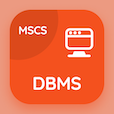Colleges Online Courses

College Math Quizzes

College Math Quiz PDF - Complete

# Trigonometric Identities Multiple Choice Questions p. 56

Study Trigonometric Identities multiple choice questions and answers, trigonometric identities quiz answers PDF 56 to study College Math course online. Trigonometric Identities MCQ trivia questions, trigonometric identities Multiple Choice Questions (MCQ) for online college degrees. "Trigonometric Identities MCQ" PDF eBook: arithmetic mean (am), when q(x) has repeated linear factors, trigonometry basics, groups in maths, trigonometric identities test prep for GRE test prep classes.

"Cosα =" MCQ PDF: 2cos²α/2 +1, 2cos²α/2 -1, 2cos²α-1, and 2cos²α+1 for SAT prep classes. Learn trigonometric identities questions and answers to improve problem solving skills for schools that offer online degrees.

## Trigonometric Identities Questions and Answers MCQs

MCQ: Cosα =

2cos²α/2 -1
2cos²α/2 +1
2cos²α-1
2cos²α+1

MCQ: If a, b are the elements of a group G, then (ba)-1 =

a-1 b-1
b-1 a-1
a-1 b
b-1 a

MCQ: Sinθ-cosθtanθ/2/cosθ+sinθtanθ/2 =

tanθ/2
−tanθ/2
cotθ/2
None of Above

MCQ: Partial fractions of 1/(x³-1) will be of the form

A/(x-1) - B/(x²+x + 1)
A/(x+1) + B/(x²+x +1)
A/(x+1) + bx + c/(x²+x + 1)
Ax+B/(x-1) + C/(x²-x + 1)

MCQ: The arithmetic mean between a and b is

a-b/2
b-a/2
a + b/2
±√(ab)

### More Quizzes from College Math Course